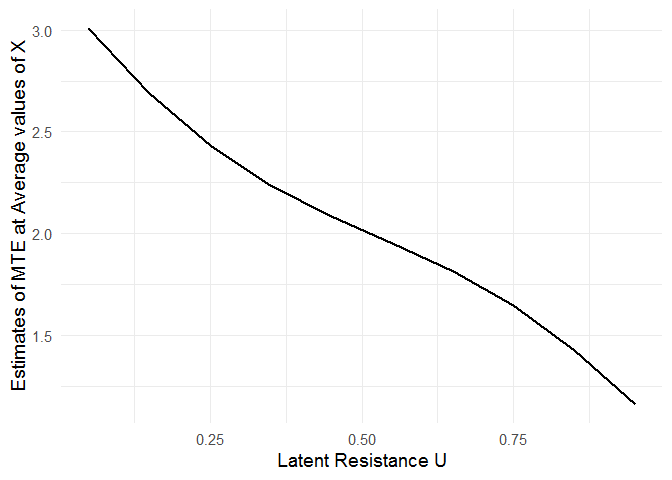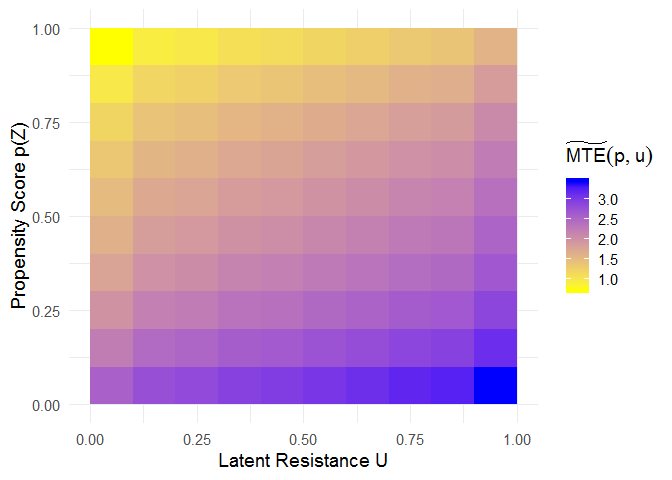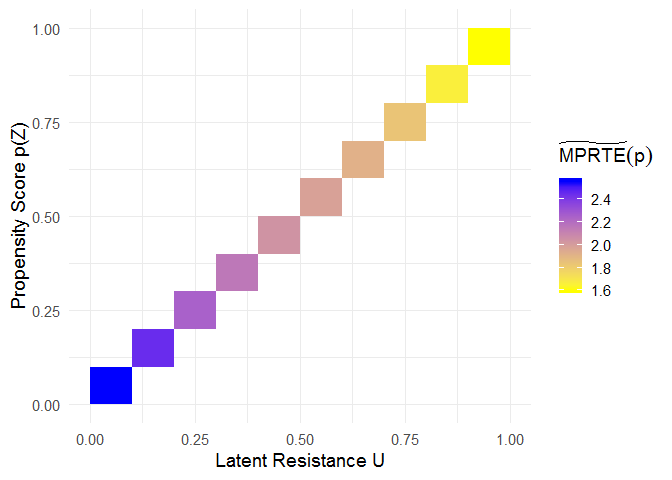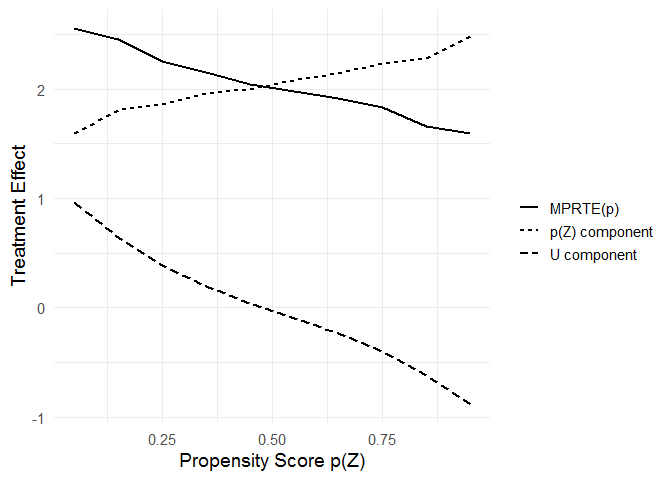# localIV: Estimation of Marginal Treatment Effects using Local Instrumental Variables

In the generalized Roy model, the marginal treatment effect (MTE) can be used as a building block for constructing conventional causal parameters such as the average treatment effect (ATE) and the average treatment effect on the treated (ATT). Given a treatment selection equation and an outcome equation, the function `mte()` estimates the MTE via the semiparametric local instrumental variables (localIV) method or the normal selection model. The function `mte_at()` evaluates MTE at different values of the latent resistance u with a given X = x, and the function `mte_tilde_at()` evaluates MTE projected onto the estimated propensity score. The function `ace()` estimates population-level average causal effects such as ATE, ATT, or the marginal policy relevant treatment effect (MPRTE).

Main References

## Installation

You can install the released version of localIV from CRAN with:

``install.packages("localIV")``

And the development version from GitHub with:

``````# install.packages("devtools")
devtools::install_github("xiangzhou09/localIV")``````

## Example

Below is a toy example illustrating the use of `mte()` to fit an MTE model using the local IV method.

``````library(localIV)

mod <- mte(selection = d ~ x + z, outcome = y ~ x, data = toydata, bw = 0.2)

# fitted propensity score model
summary(mod\$ps_model)
#>
#> Call:
#> glm(formula = selection, family = binomial("probit"), data = mf_s)
#>
#> Deviance Residuals:
#>     Min       1Q   Median       3Q      Max
#> -3.4345  -0.6555   0.0115   0.6392   3.3983
#>
#> Coefficients:
#>             Estimate Std. Error z value Pr(>|z|)
#> (Intercept)  0.01798    0.01580   1.138    0.255
#> x            0.95263    0.02065  46.124   <2e-16 ***
#> z            1.00431    0.02088  48.094   <2e-16 ***
#> ---
#> Signif. codes:  0 '***' 0.001 '**' 0.01 '*' 0.05 '.' 0.1 ' ' 1
#>
#> (Dispersion parameter for binomial family taken to be 1)
#>
#>     Null deviance: 13862.7  on 9999  degrees of freedom
#> Residual deviance:  8217.1  on 9997  degrees of freedom
#> AIC: 8223.1
#>
#> Number of Fisher Scoring iterations: 5``````

After fitting the MTE model, the `mte_at()` function can be used to examine treatment effect heterogeneity as a function of the latent resistance u.

``````
mte_vals <- mte_at(u = seq(0.05, 0.95, 0.1), model = mod)

# install.packages("ggplot2")
library(ggplot2)

ggplot(mte_vals, aes(x = u, y = value)) +
geom_line(size = 1) +
xlab("Latent Resistance U") +
ylab("Estimates of MTE at Average values of X") +
theme_minimal(base_size = 14)``````The `mte_tilde_at()` function estimates the “MTE tilde”, i.e., the expected treatment effect conditional on the propensity score p and the latent resistance u. It reveals treatment effect heterogeneity as a function of both p and u.

``````
u <- p <- seq(0.05, 0.95, 0.1)
mte_tilde <- mte_tilde_at(p, u, model = mod)

# heatmap showing MTE_tilde(p, u)
ggplot(mte_tilde\$df, aes(x = u, y = p, fill = value)) +
geom_tile() +
scale_fill_gradient(name = expression(widetilde(MTE)(p, u)), low = "yellow", high = "blue") +
xlab("Latent Resistance U") +
ylab("Propensity Score p(Z)") +
theme_minimal(base_size = 14)``````When u = p, the “MTE tilde” corresponds to the marginal policy relevant treatment effect (MPRTE) as a function of p.

``````
mprte_tilde_df <- subset(mte_tilde\$df, p == u)

# heatmap showing MPRTE_tilde(p)
ggplot(mprte_tilde_df, aes(x = u, y = p, fill = value)) +
geom_tile() +
scale_fill_gradient(name = expression(widetilde(MPRTE)(p)), low = "yellow", high = "blue") +
xlab("Latent Resistance U") +
ylab("Propensity Score p(Z)") +
theme_minimal(base_size = 14)````````````
# decomposition of MPRTE_tilde(p) into the p-component and the u-component

# install.packages(c("dplyr", "tidyr"))
library(dplyr)
library(tidyr)

mprte_tilde_df %>%
pivot_longer(cols = c(u_comp, p_comp, value)) %>%
mutate(name = recode_factor(name,
`value` = "MPRTE(p)",
`p_comp` = "p(Z) component",
`u_comp` = "U component")) %>%
ggplot(aes(x = p, y = value)) +
geom_line(aes(linetype = name), size = 1) +
scale_linetype("") +
xlab("Propensity Score p(Z)") +
ylab("Treatment Effect") +
theme_minimal(base_size = 14)``````Finally, the `ace()` function can be used to estimate population-level Average Causal Effects including ATE, ATT, ATU, and the marginal policy relevant treatment effect (MPRTE). When estimating the MPRTE at the population level, `policy` needs to be specified as an expression representing a univariate function of `p`.

``````
ate <- ace(mod, "ate")
att <- ace(mod, "att")
atu <- ace(mod, "atu")
mprte1 <- ace(mod, "mprte")
mprte2 <- ace(mod, "mprte", policy = p)
mprte3 <- ace(mod, "mprte", policy = 1-p)
mprte4 <- ace(mod, "mprte", policy = I(p<0.25))

c(ate, att, atu, mprte1, mprte2, mprte3, mprte4)
#>                ate                att                atu           mprte: 1
#>           2.045050           2.525198           1.559401           2.048361
#>           mprte: p       mprte: 1 - p mprte: I(p < 0.25)
#>           1.826024           2.272419           2.486643``````